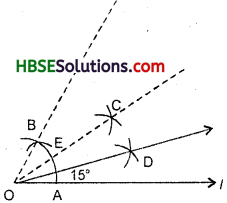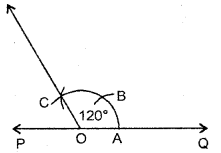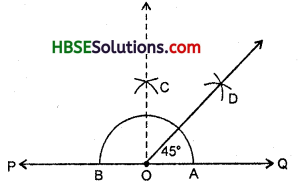# HBSE 6th Class Maths Solutions Chapter 14 Practical Geometry Intext Questions

Haryana State Board HBSE 6th Class Maths Solutions Chapter 14 Practical Geometry Ex 14.5 Textbook Exercise Questions and Answers.

## 14.5

TRY THESE :

Question 1.
In step 2 above, what would happen if we take the length of radius to
be smaller than half the length of $$\overline{\mathrm{AB}}$$ ?
Solution:
If we take the radius to be smaller than half of the length of $$\overline{\mathrm{AB}}$$, the arcs will not intersect each other at two points C and D.TRY THIS (Page 380) :

Question 1.
How will you construct a 15° angle ?
Solution:
Construct of angle an 30° as shown earlier.
Now bisect this angle. We get ∠AOD = 15° [Fig.],TRY THESE (Page 381) :

Question 1.
How will you. construct a 150° angle ?
Solution:
Construct an angle of 120° as shown earlier.
Now ∠POQ = Straight angle – 180°
∠QOC = 120°
∠POC = 180° – 120° = 60°Bisect ∠POC to get ∠COD = 30°
Thus, ∠QOD = ∠QOC + ∠COD = 120° + 30° = 150° which is the required angle.TRY THESE
Question.
How will you construct a 45° angle ?
Solution:
Construct ∠QOC = 90° as shown earlier. Raw $$\overrightarrow{\mathrm{OD}}$$ as the bisector of ∠QOC.
Thus, ∠QOD = 45° is the required angle.